### 3rd Wave in India, Covid Debacle Continues - Let us Use Covid Data to Learn Piecewise LR and Exponential Curve Fitting

Posted January 7, 2022 by Gowri Shankar  ‐  8 min read

Deep neural networks models are dominant in their job compared to any other algorithms like support vectors machines or statistical models that are celebrated once. When it comes to big data, without a doubt deep learning models are the defacto choice for convergence. I often wonder what must be making them so efficient, something should be quite obvious and provable. Activation functions, we know activation functions bring in non-linearity to the network layers through the neurons and they do the magic in vogue. ReLU, Sigmoid, and their sister-in-law gang are the piecewise linear functions that create non-linearity to the outcomes. i.e. the activation functions help the neural networks to slice and dice the input space into finer grains and form locally sensitive hash tables. A piecewise linear function in the network can be visualized as a polyhedron(or a cell) with sharp edges is the fundamental building block for achieving convergence in DNNs.

The above explanation for the success of neural networks is most convincing and thought-provoking. Certain beliefs are known, accepted but often unconvincingly because of the gap in understanding the fundamental ideas and concepts. When we attempt to ask more and more questions, we see the light - that is the process of learning. This post has 2 parts, we shall study Piecewise Linear Regression models in the first part and how to fit an exponential curve in the second one. This post falls under the section Fundamentals of AI/ML and Convergence under Math for AI/ML, previous posts under these topics can be referred to here,I thank Tim Scarfe and fearsome(with his knowledge) Dr. Keith Duggar(MIT PhD) for brilliantly explaining the concept of Activation Functions within the context of Piecewise Linear Functions in their 2022 premiere of machine learning street talk. Without the inspiration from them, this article wouldn’t have been possible.

### Objective

The objective of this post is to understand the Piecewise Linear Regression without much mathematics but through coding. We also study how to fit an exponential curve in the second section. With that quest, we cover the following,

• An explorative analysis of the Covid-19 dataset
• Univariate spline smoothing using SciKit interpolate
• PWLF: Piecewise Linear Fitting library for Piecewise Linear Regression
• Math behind logarithmic fitting

## Introduction

We are in the nascent stages of understanding how deep neural network models are bringing accurate predictions. Most of the models are not explainable and lack sufficient trust for the end-user to let the machines make the decisions. Hence, we seek models to provide recommendations with uncertainty measurements to increase their reliability. For example, the right way to find the area under a curve is to look at the curve with a magnifying lens and dissect them using a knife to make smaller rectangles and then sum the area of smaller rectangles. i.e. Divide and Conquer. The same principle is apt for fitting curves. To demonstrate this, let us load the global Covid-19 dataset and then analyze a portion of it.

Covid data used in this analysis is taken from Our World In Date: Coronavirus (COVID-19) Cases on $7^{th}$ Jan 2022, 12 PM IST

import pandas as pd
import numpy as np
import matplotlib.pyplot as plt
import seaborn as sns

#%matplotlib inline

covid_df = pd.read_csv("./owid-covid-data.csv", sep=",", parse_dates=["date"])
print(f"No of features: {covid_df.shape}, No of observations: {covid_df.shape}")
print(f"Start Date: {min(covid_df.date)}, End Date: {max(covid_df.date)}")

No of features: 67, No of observations: 152691
Start Date: 2020-01-01 00:00:00, End Date: 2022-01-06 00:00:00

covid_ind_df = covid_df[covid_df.location == "India"]
covid_ind_df.iloc[:, 0:10].tail()


iso_codecontinentlocationdatetotal_casesnew_casesnew_cases_smoothedtotal_deathsnew_deathsnew_deaths_smoothed
64766INDAsiaIndia2022-01-0234922882.033750.018507.000481893.0123.0270.857
64767INDAsiaIndia2022-01-0334960261.037379.022938.571482017.0124.0246.714
64768INDAsiaIndia2022-01-0435018358.058097.029924.571482551.0534.0279.857
64769INDAsiaIndia2022-01-0535109286.090928.041035.143482876.0325.0288.000
64770INDAsiaIndia2022-01-0635109286.0NaNNaN482876.0NaNNaN

### Covid Diagnosis and Growth Curves

Our goal is to fit the new covid cases(Diagnosis) and the total number of covid cases(Growth) curves under a constrained environment to understand Piecewise Linear Regression. We also fit a Logarithmic curve on the growth curve focusing on the period where the first wave ends and up to the peak of the second wave. Waves often result in exponential growth, hence we choose a logarithmic fitting scheme.

def plot_daily_cases(data, scatter=False, predictions_daily=[], predictions_total=[], titleA="", titleB="", sup_title=""):
fig, axes = plt.subplots(1, 2, figsize=(25, 6))
if(scatter):
axes.scatter(data.date.values, data.new_cases.values, label='Observed')
axes.scatter(data.date.values, data.total_cases.values, label='Observed')
else:
axes.plot(data.date.values, data.new_cases.values, label='Observed')
axes.plot(data.date.values, data.total_cases.values, label='Observed')
if(len(predictions_daily) == len(data)):
axes.plot(data.date.values, predictions_daily, color="red", linestyle='dashed',
linewidth=5, label='Predicted')

if(len(predictions_total) == len(data)):
axes.plot(data.date.values, predictions_total, color="red", linestyle='dashed',
linewidth=5, label='Predicted')
axes.legend()
axes.legend()
axes.set_title(titleA)
axes.set_title(titleB)

fig.suptitle(sup_title, fontsize=20)

plt.show()

from scipy.interpolate import UnivariateSpline
def smooth_spline(data, sm_factor=0.5):
spl = UnivariateSpline(np.arange(len(data)), data, s=len(data))
spl.set_smoothing_factor(sm_factor)
return spl(data)

plot_daily_cases(
covid_ind_df,
titleA="Daily - No. of Individuals Diagnosed +ve",
titleB="Growth - Total No of Infected Individuals",
sup_title="Covid Cases(India)"
)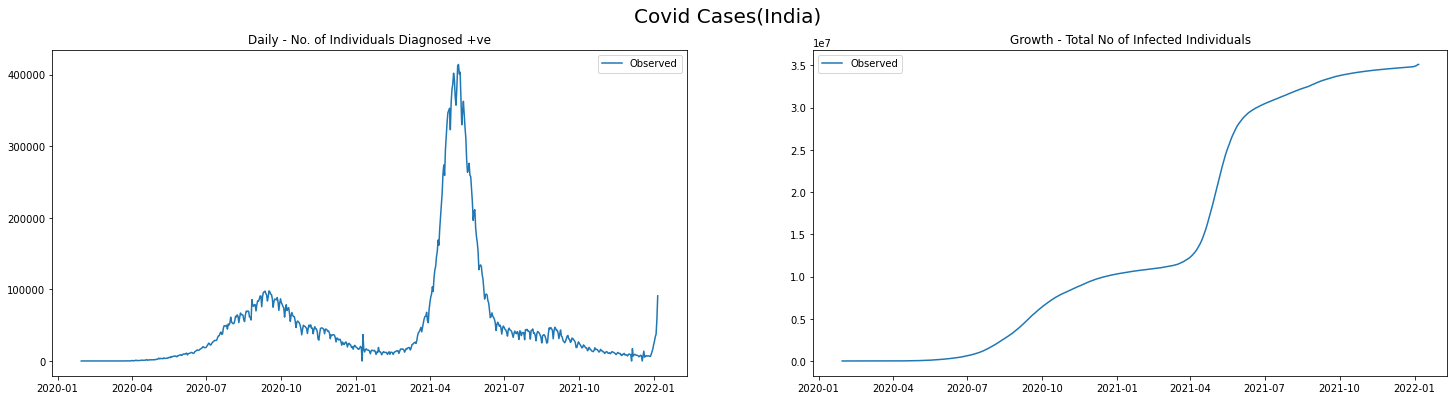### Data Subset

Few observations from the data

• We see there are 2 peaks and a shooting count at the tail end - i.e 2 completed waves and the 3rd is about to start
• The first wave subsided around September of 2020 - If I remember correctly, August 2020 was the month Amazon started delivering non-essential goods
• The second wave started by March 2021

We shall extract the covid new cases and total diagnosed data between October 2020 and March 2021 for understanding Piecewise Linear Regression.

I used univariate spline fit to smooth the data to simplify things

covid_SEP20_MAR21_df = covid_ind_df[(covid_ind_df.date >= "2020-10-01") & (covid_ind_df.date <= "2021-03-30")]
plot_daily_cases(
covid_SEP20_MAR21_df,
scatter=True,
titleA="Daily - No. of Individuals Diagnosed +ve",
titleB="Growth - Total No of Infected Individuals",
sup_title="Covid Cases(India) $1^{st}$ Oct, 2020 - $30^{th}$ Mar, 2021"
)

smoothened_data = smooth_spline(covid_SEP20_MAR21_df.new_cases.values, sm_factor=0.1)
covid_SEP20_MAR21_df.loc[:, "new_cases"] = smoothened_data
plot_daily_cases(
covid_SEP20_MAR21_df,
scatter=True,
titleA="Daily - No. of Individuals Diagnosed +ve(Smooth)",
titleB="Growth - Total No of Infected Individuals",
sup_title="Covid Cases(India) $1^{st}$ Oct, 2020 - $30^{th}$ Mar, 2021"
)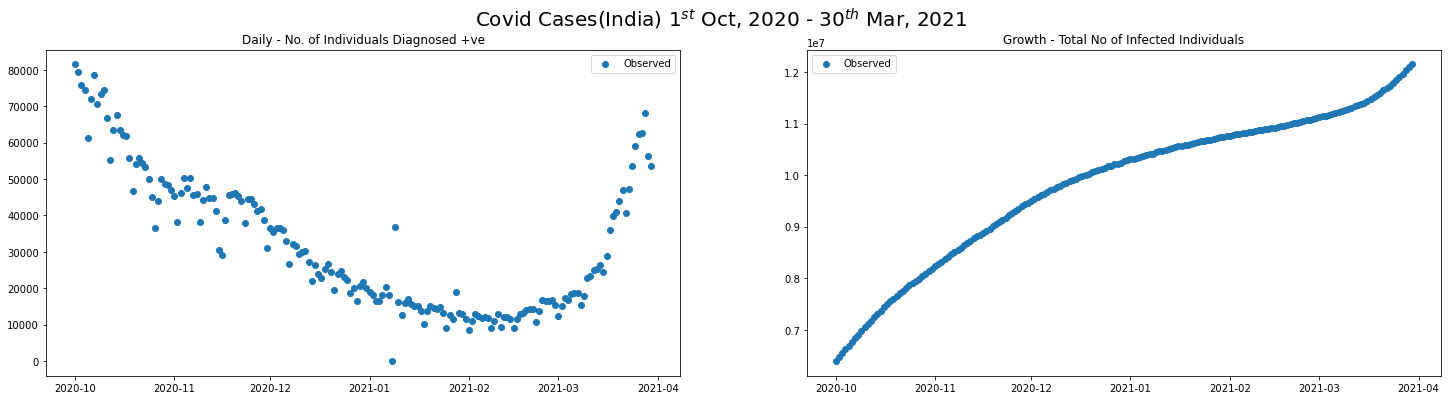/Users/shankar/dev/tools/anaconda3/envs/nxt/lib/python3.9/site-packages/pandas/core/indexing.py:1773: SettingWithCopyWarning:
A value is trying to be set on a copy of a slice from a DataFrame.
Try using .loc[row_indexer,col_indexer] = value instead

See the caveats in the documentation: https://pandas.pydata.org/pandas-docs/stable/user_guide/indexing.html#returning-a-view-versus-a-copy
self._setitem_single_column(ilocs, value, pi)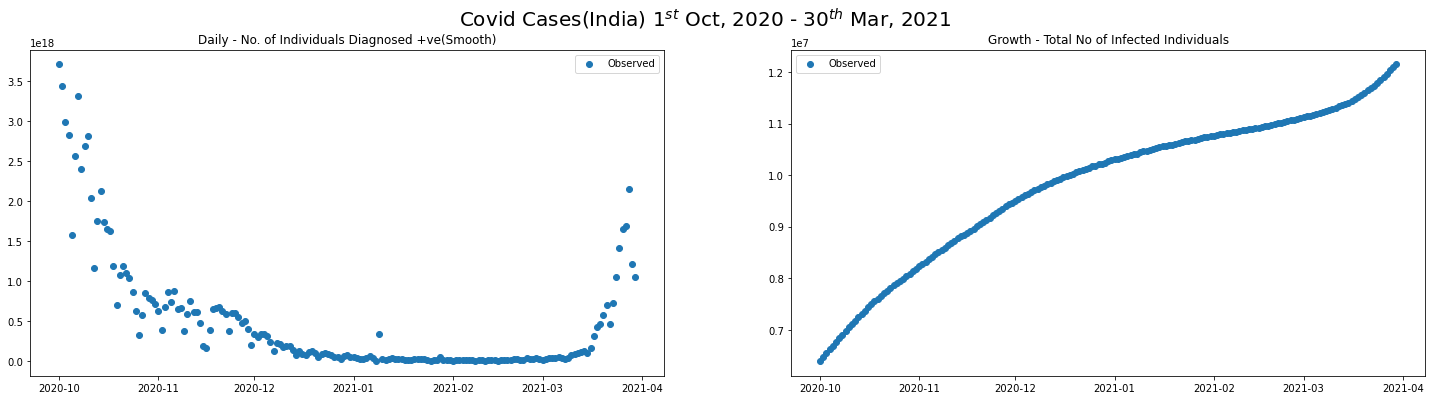### Observe in Pieces

From the graphs we can see, there are 6 different regions where trend shifts during the period between the end of the first wave and the beginning of the second wave.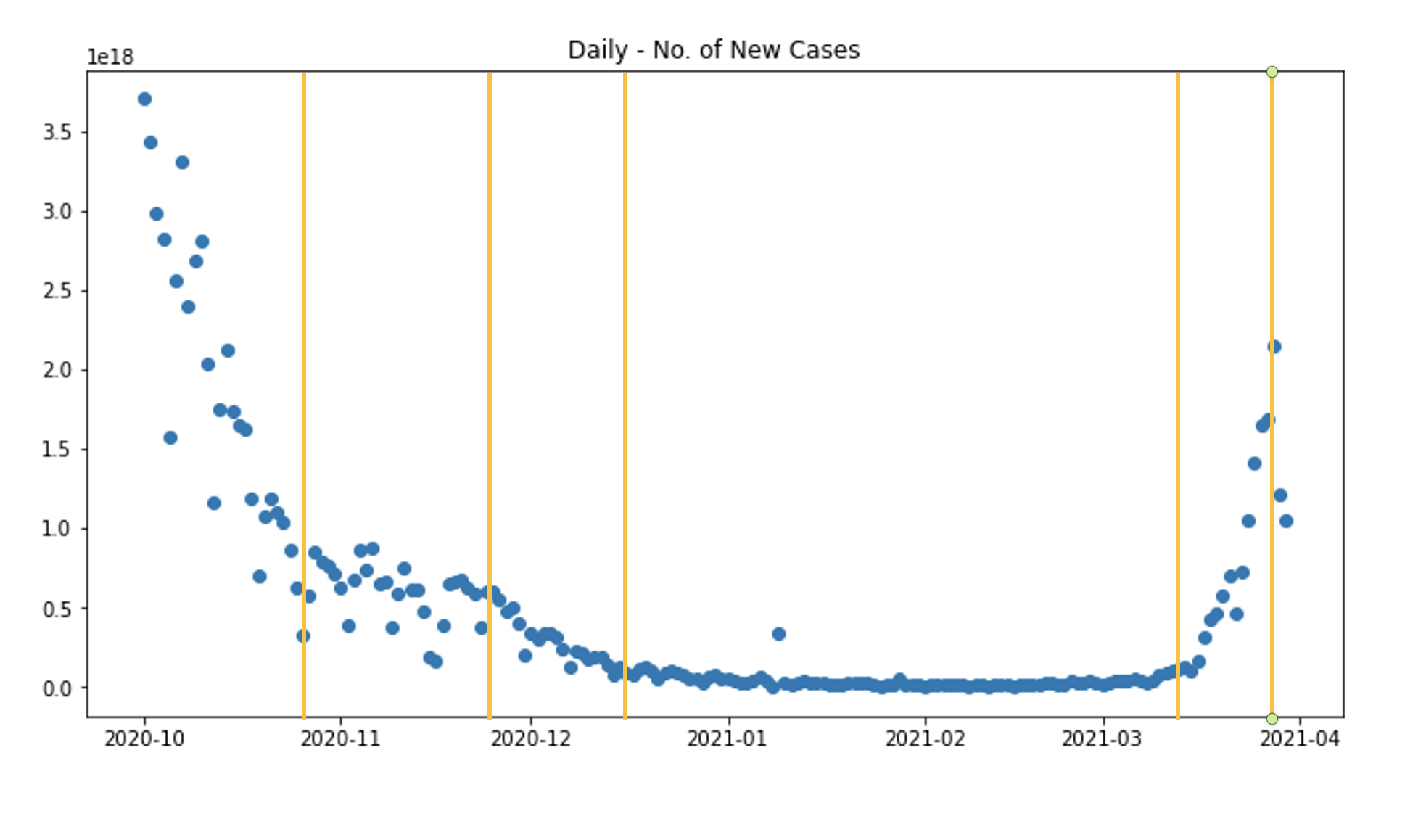# !python -m pip install pwlf


### Piecewise Linear Regression

We need Piecewise Linear Regression models when the relationship between the dependent variable$(y)$ and the independent variable$(x)$ is continuous and consist of 2 or more linear segments. Then such function is estimated using nonlinear least squares by specifying the number of segments. Let us say the model fit consist of $k$ linear segment(in our example it is 6) then, $$\Large y = \beta_0 + \beta_1 x + \sum_{j=1}^{k-1} \beta_{j+1}(x - \Delta_j)I(x - \Delta_j) \tag{1. Piecewise Linear Regression Model}$$ Where,

• ${\beta_0}$ is the $y$-intercept
• ${\beta_j}$ is the slope of the segment, ${j=1,2,3, \cdots, k}$
• $\Delta_j$ location of the slope changes between segment $j$ and segment $j+1$
• $I(x-\Delta_j) = 1 \ if \ x \geq \Delta_j$ and $0$ otherwise
import pwlf
pwlf_model = pwlf.PiecewiseLinFit(np.arange(len(covid_SEP20_MAR21_df)), covid_SEP20_MAR21_df.new_cases.values)
z = pwlf_model.fit(5)
slopes = pwlf_model.calc_slopes()

# Predictions
predicted_new_cases = pwlf_model.predict(np.arange(len(covid_SEP20_MAR21_df)))

/Users/shankar/dev/tools/anaconda3/envs/nxt/lib/python3.9/site-packages/pwlf/pwlf.py:1086: RuntimeWarning: invalid value encountered in true_divide
self.slopes = np.divide(

plot_daily_cases(
covid_SEP20_MAR21_df,
scatter=True,
predictions_daily=predicted_new_cases,
titleA="Daily - No. of Individuals Diagnosed +ve(Smooth)",
titleB="Growth - Total No of Infected Individuals",
sup_title="Covid Cases(India) $1^{st}$ Oct, 2020 - $30^{th}$ Mar, 2021 Actual vs Predictions"
)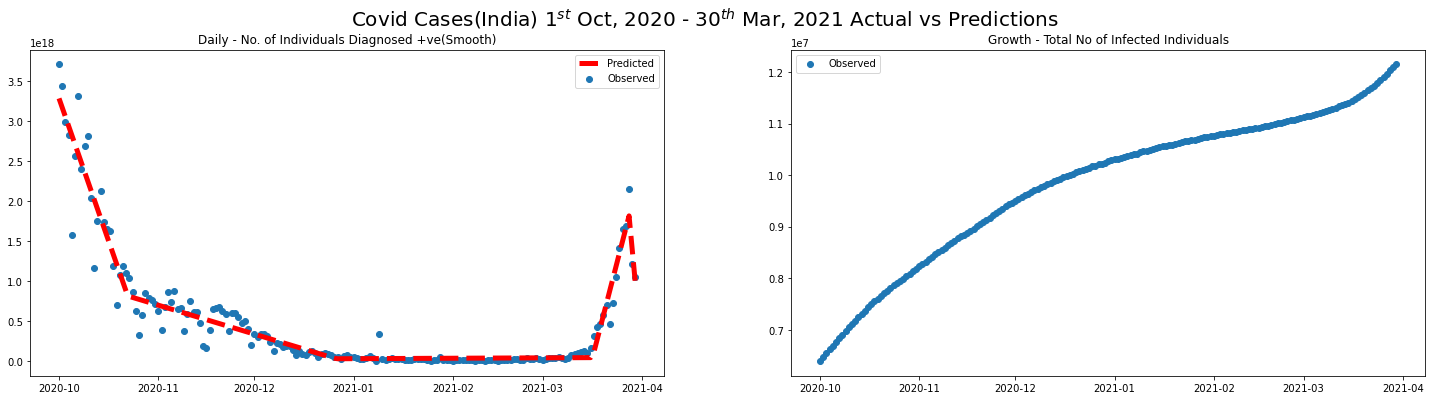## Exponential and Logarithmic Curve Fitting

We know a simple linear regression model is represented as $y = Ax + B$ and we have studied the Piecewise Linear Regression model in the previous section. An exponential curve fitting model is represented as follows,

$$\Large y = Ae^{Bx} \tag{2. An Exponential Model}$$ $$take \ log \ on \ both \ sides$$ $$log(y) = log(Ae^{Bx}) = log(A) + log(e^{Bx}))$$ $$log(y) = log(A) + Bx$$

Let us do the following,

• Subset the data for a period where there is an exponential growth
• Fit the model using numpy.polyfit function
• Apply piecewise linear regression for the log(y)

Yes, It is a hack but the intent of this post is to understand the idea behind the Piecewise approach and nothing beyond

covid_2nd_wave_df = covid_ind_df[(covid_ind_df.date >= "2021-01-31") & (covid_ind_df.date <= "2021-05-10")]
plot_daily_cases(
covid_2nd_wave_df,
scatter=True,
titleA="Daily - No. of Individuals Diagnosed +ve",
titleB="Growth - Total No of Infected Individuals",
sup_title="Covid Cases(India) $31^{st}$ Jan, 2021 - $10^{th}$ May, 2021"

)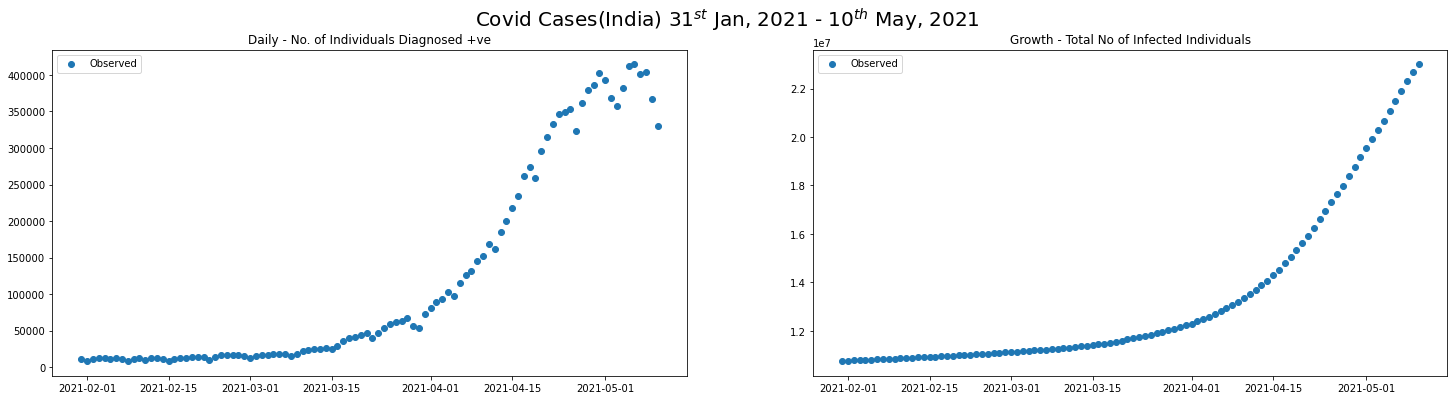NUM_PIECES = 10
def logarithmic_fit(data):
prediction_coeffs = np.polyfit(
np.arange(len(data)),
np.log(data.total_cases),
1,
w= np.sqrt(data.total_cases)
)
B, A = prediction_coeffs

preds = [np.exp(A) * np.exp(B * x) for x in np.arange(len(data))]

return preds

def piecewise_logarithmic_fit(data, num_pieces=NUM_PIECES):

piece_length = len(data) // num_pieces

predictions = []
for i in np.arange(num_pieces):
offset = i * piece_length
piece_of_data = data[offset:offset + piece_length ]
preds = logarithmic_fit(piece_of_data)
predictions.append(preds)

predictions = np.concatenate(predictions)

return predictions


preds = logarithmic_fit(covid_2nd_wave_df)
plot_daily_cases(
covid_2nd_wave_df,
scatter=True,
predictions_total=preds,
titleA="Daily - No. of Individuals Diagnosed +ve",
titleB="Growth - Total No of Infected Individuals",
sup_title="Covid Cases(India) $31^{st}$ Jan, 2021 - $10^{th}$ May, 2021 Actual vs Predictions"
)

pwlf_model = pwlf.PiecewiseLinFit(np.arange(len(covid_2nd_wave_df)), covid_2nd_wave_df.new_cases.values)
z = pwlf_model.fit(5)
slopes = pwlf_model.calc_slopes()

# Predictions
predicted_new_cases = pwlf_model.predict(np.arange(len(covid_2nd_wave_df)))

preds = piecewise_logarithmic_fit(covid_2nd_wave_df)
plot_daily_cases(
covid_2nd_wave_df,
scatter=True,
predictions_daily=predicted_new_cases,
predictions_total=preds,
titleA="Daily - No. of Individuals Diagnosed +ve",
titleB="Growth - Total No of Infected Individuals",
sup_title="Covid Cases(India) $31^{st}$ Jan, 2021 - $10^{th}$ May, 2021 Actual vs Piecewise Predictions"
)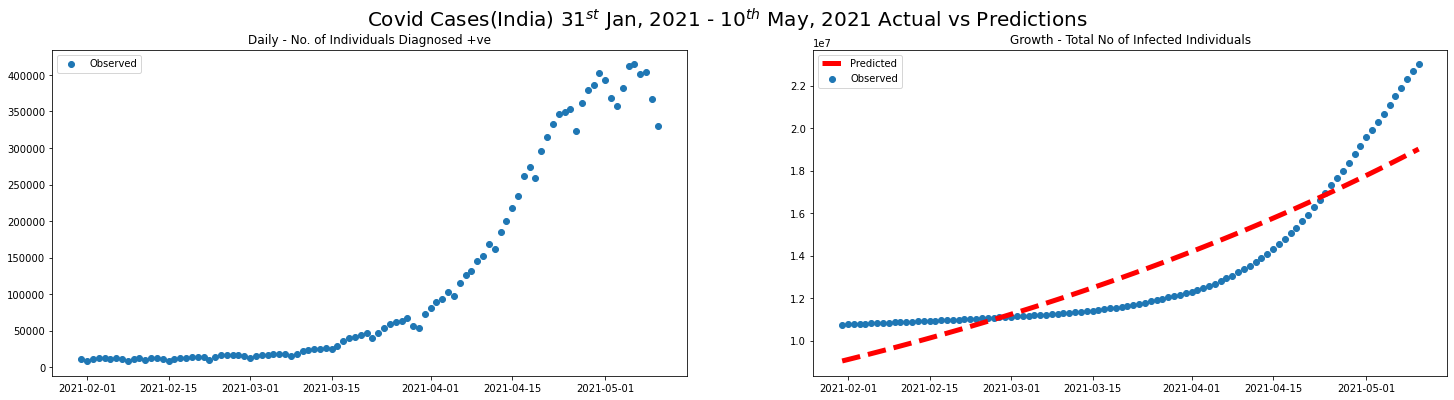/Users/shankar/dev/tools/anaconda3/envs/nxt/lib/python3.9/site-packages/pwlf/pwlf.py:1086: RuntimeWarning: invalid value encountered in true_divide
self.slopes = np.divide(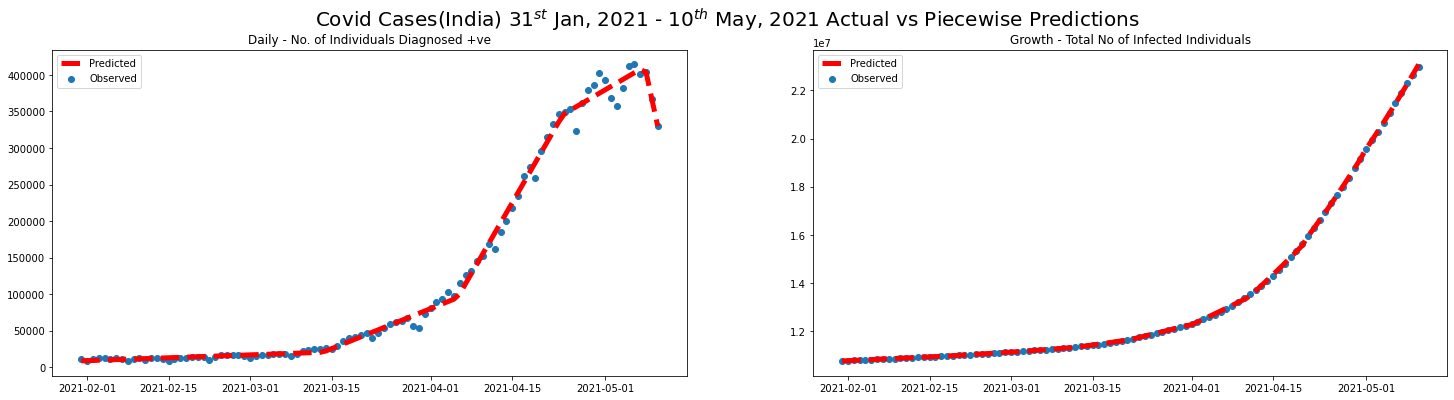## Epilogue

In this post, we took one of the most fundamental concepts of machine learning and analyzed using the covid data. Our goal was to learn how the piecewise approach works to get an intuition of polyhedron(cell) in the complex and large-scale DNNs. I took many shortcuts, assumptions, and presumptions to make this post concise and precise at the expense of accuracy/correctness - I request the readers to pardon me for this approach. In my defense, complete learning and understanding a complex concept is a continuous process because we all are in different stages of comprehension. Hoping this post is a useful one that declutters a few critical ideas.

3rd-wave-in-india-covid-debacle-continues-let-us-use-covid-data-to-learn-piecewise-lr-and-exponential-curve-fitting